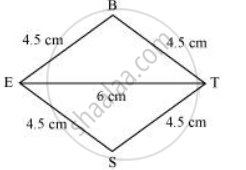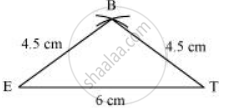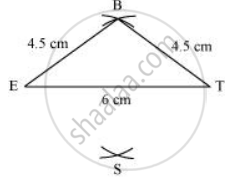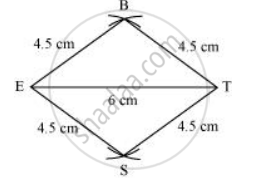Share

# Construct the Following Quadrilaterals. Rhombus Best Be = 4.5 Cm Et = 6 Cm - Mathematics

Course
ConceptConstructing a Quadrilateral When the Lengths of Four Sides and a Diagonal Are Given

Rhombus BEST

BE = 4.5 cm

ET = 6 cm

#### Solution

We know that all sides of a rhombus are of the same measure.

Hence, BE = ES = ST = TB

A rough sketch of this rhombus can be drawn as follows.1) Δ BET can be constructed by using the given measurements as follows.2) Vertex S is 4.5 cm away from vertex E and also from vertex T. Therefore, while taking E and T as centres, draw arcs of 4.5 cm radius, which will be intersecting each other at point S.3) Join S to E and T.BEST is the required rhombus.

Is there an error in this question or solution?

#### APPEARS IN

NCERT Solution for Mathematics Textbook for Class 8 (2018 to Current)
Chapter 4: Practical Geometry
Ex. 4.1 | Q: 1.4 | Page no. 60

#### Video TutorialsVIEW ALL 

Solution Construct the Following Quadrilaterals. Rhombus Best Be = 4.5 Cm Et = 6 Cm Concept: Constructing a Quadrilateral - When the Lengths of Four Sides and a Diagonal Are Given.
S